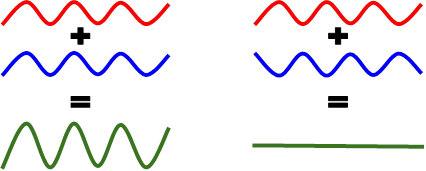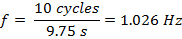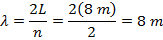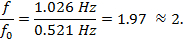Trial ends in

A subscription to JoVE is required to view this content.
You will only be able to see the first 20 seconds.

Standing Waves
Inductance
Science Education (Physics II)
Reflection and Refraction

Standing Waves

Overview

Source: Arianna Brown, Asantha Cooray, PhD, Department of Physics & Astronomy, School of Physical Sciences, University of California, Irvine, CA

Standing waves, or stationary waves, are waves that appear not to propagate and are produced by the interference of two waves traveling in opposite directions with the same frequency and amplitude. These waves appear to vibrate up and down with no linear movement and are most easily identified in vibrating finite media like a plucked guitar string, water in a lake, or air in a room. For example, if a string is fixed at both ends and two identical waves are sent traveling along the length, the first wave will hit the end barrier and reflect back in the opposite direction, and the two waves will superpose to produce a standing wave. This motion is periodic with frequencies defined by the length of the medium and is a visual example of simple harmonic motion. Simple harmonic motion is motion that oscillates or is periodic, where the restoring force is proportional to the displacement, meaning the farther something is pushed, the harder it pushes back.

The goal of this experiment is to understand the roles of wave superposition and reflection in creating standing waves, and exploit those concepts to calculate the first few resonant frequencies, or harmonics, of standing waves on a slinky. Each frequency that an object produces has its own standing wave patterns, where the wave with the lowest possible frequency is called the fundamental frequency. A harmonic is a wave that has a frequency proportionate to the fundamental frequency by whole integer numbers.

Principles

When two waves arrive at the same point in a path at the same time, they interfere. The amplitude of the resulting wave is the sum of the amplitude of the two linear waves (a direct result of the principle of superposition for linear waves). These two waves pass through one another without altering each other's paths or speeds. Constructive interference occurs when the amplitudes of the waves add, as they arrive in phase. When waves meet out of phase, their amplitudes subtract and they undergo destructive interference. If two waves with the same amplitude undergo destructive interference, their amplitudes cancel (Figure 1).Figure 1: Two waves with equal amplitudes. Left: Constructive interference. Right: Destructive interference.

When a traveling wave meets a boundary (i.e., a different medium), some of its energy is reflected, some is transmitted into the new medium, and some is absorbed. For a perfect reflected wave scenario, where all of the energy is reflected and so no outside energy needs to be fed into the system, the energy is conserved. For a wave traveling on a medium with fixed boundaries, like a finite string, it will reflect off the end boundary and experience a 180 ° phase shift. If this process continues for an extended period of time, the waves bouncing back and forth between boundaries will interfere and create a stationary pattern known as a standing wave (Figure 2). The points of minimum amplitude (nodes) are points where the waves have opposite phases and cancel one another out. The points of maximum amplitude (antinodes) are points where the waves have the same phase and their respective amplitudes combine.Figure 2: A standing wave on a medium of length 2λ. This is also a visual representation of the fourth harmonic.

The simplest standing wave, sometimes called the fundamental frequency, occurs when the length of the string L is ½λ, where λ is the wavelength. This means,so the first vibration on a string with fixed points looks similar to the profile of a jump rope in motion. To make the next possible standing wave, a node is added in the center, and L becomes equivalent to λ: the result is a standing wave pattern with a shorter wavelength. Standing wave patterns with shorter wavelengths than the fundamental frequency are known as harmonics. Continuing to add nodes, we find that:(Equation 1)

where n is the number of nodes and the resulting harmonic is sometimes referred to as the nth harmonic. (Note: Some refer to the fundamental frequency as the first harmonic, while others refer to the n = 2 harmonic as the first harmonic).

In a propagating wave, energy is transferred along with the wave. As one section moves upward, it exerts a force on the next section, moving it through a displacement. In other words, work is done. Points that experience no displacement, like a node in a standing wave, cannot do work on the neighboring section. Thus, no energy is transmitted across a node on a string, and energy does not propagate in a standing wave. Instead, the energy of a standing wave alternates between elastic potential energy when the waves are momentarily stationary at their maximum amplitudes, and kinetic energy when the string is flat in the middle of an oscillation and a particle on an antinode has maximum directional speed. Additionally, consider a particle that is situated on a piece of string undergoing standing wave motion. Since standing waves result in simple back and forth motion, this particle appears to move back and forth at a measureable periodic rate. In standing waves, this oscillatory motion and the relationship between elastic and potential energy is described as simple harmonic motion, and thus has the observable properties of frequency f and period T. In the standing wave scenario, frequency is defined as the number of oscillation cycles per unit time, and period is the time required to make one complete cycle, or:(Equation 2)

In this lab, we will explore all of these properties by creating various waves and standing waves using a slinky.

Procedure

1. Observing the Superposition and Reflection of Slinky Pulses

1. Stretch a slinky or steel spring lengthwise across a floor or hallway, with one student holding one end and another student holding the other. Use tape to mark two lengthwise 'barriers' about a foot away from the middle of the slinky, on each side. Repeat with barriers that are two feet away from the middle on each side.
2. Take turns launching pulses (jerking the slinky a small distance horizontally and immediately snapping back to the starting point) with amplitudes that stay within the marked barriers.
3. Next, try launching identical pulses with the same polarity simultaneously from both ends and notice what happens when the pulses meet. The superimposed wave should double in amplitude, cross the first taped barriers, and hit the second taped barriers.
4. Now, launch identical pulses but with opposite polarity simultaneously and observe the pulse superpositions. The pulses should cancel one another out as they superimpose, and then continue traveling, never touching the barriers.
5. Fix one end of the slinky by holding it tightly in position. Send a single pulse down to the fixed position and observe the wave's amplitude upon reflection. It will reflect back with opposite polarity.

2. Measuring the Frequency of Standing Waves on a Spring

1. Stretch the slinky across a room or hallway and measure and record the stretched length.
2. With one end fixed from motion (held tightly), gently begin sliding the other end horizontally in consistent motion until finding the fundamental frequency standing wave. For this harmonic, there should be only one wave crest with one amplitude moving back and forth, like the profile of a jump rope in motion. Use a stopwatch to record the time it takes for several wave cycles. One complete cycle starts when an antinode forms on one side, slides through the center to form an antinode on the other side, and then returns to its original position. Use these measurements to calculate the frequency, period, and wavelength for this wave using Equations 1 and 2.
3. Increase the speed of the sliding end until the next harmonic (n = 2) is achieved. For this harmonic, there should be two wave crests on opposite sides moving in opposite directions, and it may look like the 2D projection of the letter 's' rotating. Measure the frequency, then calculate the period and wavelength for this wave. What is the ratio of this frequency to the fundamental frequency?
4. Repeat the previous step for the next harmonic (n = 3).

Standing waves, or stationary waves, are waves that appear to not propagate and they are most evident in a vibration. For example, when a taut string is plucked, the resulting waves appear to vibrate up and down, with no linear movement. These are actually produced by the interference of two waves traveling in opposite directions, with the same frequency and amplitude.

This oscillating motion with periodic frequency is an example of simple harmonic motion. The motion happens because the string has a restoring force that is proportional to the initial displacement. This relationship between restoring force and displacement is given by Hooke's Law -- explained in detail in another JoVE Science Education video. This essentially means that the harder something is pulled, like this sling shot, the harder it pushes back.

In this video, we will create standing waves using a slinky, and explore the physics behind simple harmonic motion and its applications.

Before we start the demonstration in the laboratory, let's learn a bit more about standing waves and simple harmonic motion. A wave is defined by its wavelength, lambda -- the distance between two crests, and its frequency, f -- the number of occurrences of crests in unit time, The amplitude is the distance from crest to trough. When two waves arrive at the same point in a path, at the same time, they interfere. The amplitude of the resulting wave is the sum of the amplitudes of the two waves.

Constructive interference occurs when the amplitudes of the waves are in phase, and add. Destructive interference occurs when the waves are out of phase, and the amplitudes subtract.

Take for example, a pulse on a finite string. Ideally, when the traveling pulse meets a boundary, it is reflected. Now let's send a wave down the string, and let it reflect back and forth for an extended period of time. This action creates a stationary pattern, or standing wave.

The points of minimum amplitude, called nodes, are where the waves have opposite phases and cancel each other out. The points of maximum amplitude, or antinodes, are points where the waves have the same phase and their amplitudes combine. The simplest standing wave occurs when the wavelength is twice the length of the string.

The next possible standing wave has a node in the center, and the wavelength equals the length of the string. If we continue to add nodes, we create waves with shorter and shorter wavelengths. These patterns are called harmonics, where the number of antinodes, denoted by letter n, gives the wave of nth harmonic. So if the wave has four antinodes, the wave is the fourth harmonic.

Based on the relationship between the wavelength and the length of the string of each harmonic, we can derive a formula relating these three terms and say that lambda of an nth harmonic standing wave equals two times the length of the string divided by n.

Since 2L is the wavelength of the first harmonic, the wavelength of each harmonic is Λ1 divided by n. Now, we know that Λ and f have inverse relationship. Hence, we can deduce that the frequency of each harmonic would be the nth multiple of the first harmonic, or the ratio of the frequency to the frequency of first harmonic yields n. Note that the first harmonic is also known as the fundamental frequency of that string.

Now that we've discussed the basics of simple harmonics, let's take a look at how to make standing waves using a slinky, and how to measure the frequency of standing waves.

First, stretch a slinky or steel spring lengthwise across the floor with one person holding each end. Use tape to mark two lengthwise barriers, each about a foot away from the middle of the slinky, on each side.

Also, add lengthwise barriers that are two feet away from the middle of the slinky on each side.

Take turns for launching wave pulses by jerking the slinky a small distance horizontally, and then immediately snapping it back to the starting point. Ensure that the amplitudes stay within the marked barriers.

Next, simultaneously launch identical pulses with the same polarity, and observe what happens when the pulses meet. The superimposed wave should double in amplitude, cross the first taped barriers and hit the second taped barriers.

Now, simultaneously launch identical pulses with opposite polarity. The pulses should cancel one another out as they superimpose and continue traveling. They should never reach the barriers.

Finally, fix one end by holding it tightly in position. Send a single pulse down to the fixed position, and observe the waves amplitude as it is reflected. It will reflect back with opposite polarity.

Now let's take a look at how to measure the frequency of standing waves. Stretch the slinky across the room again, and measure the stretched length.

With one end fixed, gently begin sliding the other end horizontally until you find the first harmonic. For this harmonic, there should be only one wave's crest with one amplitude moving back and forth.

Use a stopwatch to record the time it takes for each wave cycle. One complete cycle starts when an antinode forms on one side, slides through the center to form an antinode on the other side, and then returns to the original position.

Now, increase the speed of the sliding until you reach the next harmonic. For the second harmonic, there should be two wave crests on opposite sides moving in opposite directions. Measure the time for one wave cycle.

Repeat these steps for the third harmonic.

Now that we have discussed the experiment, let's learn how the analyze the data collected in order to obtain the frequencies of different harmonics. Recall, the wavelength is equal to two times the length of the slinky divided by n. Thus, for the second harmonic, the wavelength is the length of the slinky, or 8 m.

Frequency is defined as the number of cycles per unit time. Thus, frequency can be calculated for each harmonic by dividing the number of cycles by the total time. It is evident that, as n increases, the frequency of the wave also increases.

This was noticeable during the experiment as well. Now let's verify the relationship between the frequencies and n. If we divide the frequency of each harmonic with the fundamental frequency, then we obtain these values. These values demonstrate that the second harmonic is approximately twice the frequency of the fundamental frequency and the third harmonic is thrice the fundamental frequency. Together, these results validate the harmonics formulae.

Standing waves can be found in many real world examples in science and nature.

A plucked guitar string is a simple example of a standing wave. A plucked string emits a particular sound frequency depending on the string length and how taut or dense the string is.

Each string only makes certain notes because only certain standing waves are able to form on that string. These standing waves are all integer multiples of the string's fundamental frequency. The musician can shorten the string length, creating a new set of harmonics.

Acoustophoresis, which means migration with sound, is a technique in biomedical engineering that uses standing waves to displace particles in a microscale channel of flowing liquid. This is typically performed in a microfluidic device, which has micrometer scale fluid channels.

When a standing wave with specific frequency is formed within the channel, which focuses the particles into a controlled stream. Using this method, a researcher can rapidly focus or separate microscopic entities.

You've just watched JoVE's introduction to standing waves and simple harmonic motion. You should now understand the properties of standing waves, and where they are present in every day applications. Thanks for watching!

Results

 Harmonic (n) # Cycles Total Time (s) Frequency (Hz) f/f0 Period (s) Wavelength (m) 1 10 19.2 0.521 (f0) 1 1.210 16 m 2 10 9.75 1.026 1.97 0.975 8 m 3 10 6.21 1.601 3.07 0.625 5.33 m

Table 1: Section 2 - Stretched Spring Length = 8 m

In Section 1, principles of wave superposition and reflection in a finite medium are demonstrated and confirmed as pulses were sent down the length of the slinky. Specifically, we see that when two waves with identical amplitudes and phase meet, they undergo constructive interference and their amplitudes add. Similarly, we see that when two waves with opposite polarity (180 ° phase shift) and identical amplitudes meet, they undergo destructive interference and their amplitudes cancel. The latter of these principles is key in understanding standing wave patterns.

In Section 2, the nodes and antinodes of the slinky were easily visible at various frequencies. As the number of nodes increased, so did the frequency. The wavelength is inversely proportional to frequency, so there is naturally a decrease in wavelength. The frequencies of the harmonics are positive integer multiples of the fundamental frequency that correspond to n. For example, using the n = 2 harmonic, the frequency is measured and defined as the number of cycles per unit time:The period is defined as the inverse of the frequency (Equation 2) and is equal to:The wavelength is defined in Equation 1 as:Finally, we can see the integer-proportional relationship between the harmonics and the fundamental frequency by calculating:Applications and Summary

In this experiment, the concepts of wave superposition and standing waves were explored in two demonstrations. Wave reflection and constructive versus destructive interference were visualized in the first demonstration. In the second, the changes in frequency and period were measured and higher harmonic frequencies were found to be integer multiples of the fundamental frequency.

A famous example of standing waves in the real world are the strings on a guitar, or any string instrument. In these instruments, a plucked string emits a particular frequency depending on how taut and dense the string is and the string length. Each string only makes certain notes because only certain standing waves, or harmonics, can form on that string. The musician can use their fingers to shorten the string length, creating a new node and new set of harmonics that are proportional to the fundamental frequency. Vibrations that are not at the right frequency, say fingers pinning the strings down onto a fret that doesn't allow for a standing wave at that string length, will sound odd and eventually cancel themselves out.

Standing waves also occur in nature, often in bounded bodies of water like lakes and harbors. Sometimes, they can form on a river bed allowing river surfers to ride this wave for an extended period of time without actually moving. Typically, they form when a large amount of water is flowing over an obstruction, like a big rock, at a rapid pace. As the water flows over the rock and crashes behind it, it creates a large wave in the opposite direction of the river's current that interferes with the incident wave of water. Thus, a standing wave is formed and river surfers can ride it for as long as their balance will allow them since the wave likely will not end in a matter of seconds.X